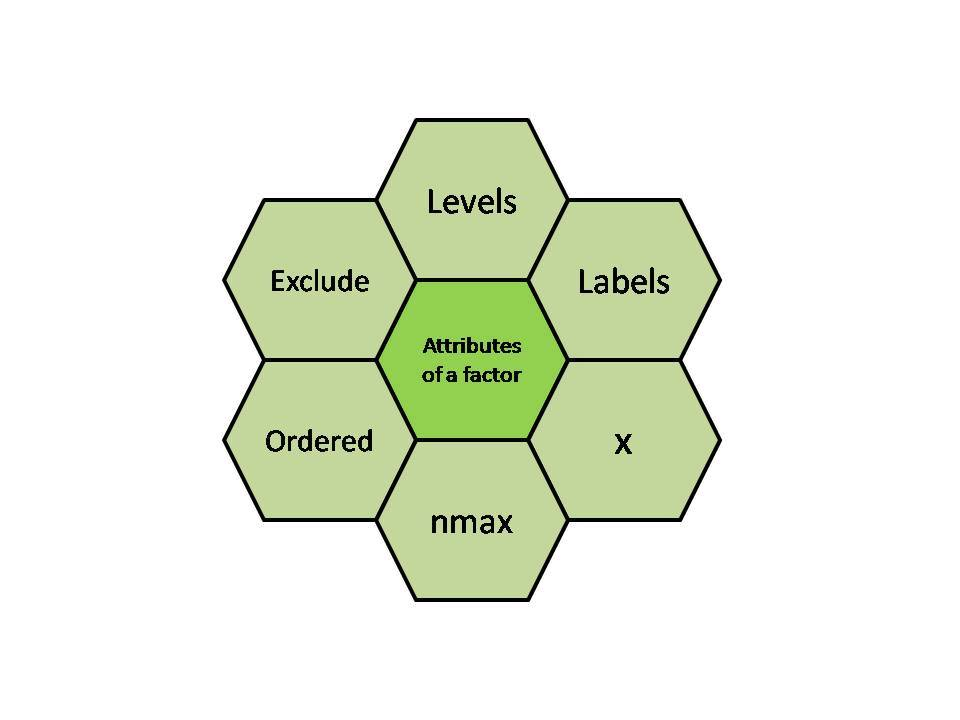GFG App
Open AppBrowser
Continue

# R Factors

Factors in R Programming Language are data structures that are implemented to categorize the data or represent categorical data and store it on multiple levels.

They can be stored as integers with a corresponding label to every unique integer. The R factors may look similar to character vectors, they are integers and care must be taken while using them as strings. The R factor accepts only a restricted number of distinct values. For example, a data field such as gender may contain values only from female, male, or transgender.

In the above example, all the possible cases are known beforehand and are predefined. These distinct values are known as levels. After a factor is created it only consists of levels that are by default sorted alphabetically.

## Attributes of Factors in R Language

• x: It is the vector that needs to be converted into a factor.
• Levels: It is a set of distinct values which are given to the input vector x.
• Labels: It is a character vector corresponding to the number of labels.
• Exclude: This will mention all the values you want to exclude.
• Ordered: This logical attribute decides whether the levels are ordered.
• nmax: It will decide the upper limit for the maximum number of levels.## Creating a Factor in R Programming Language

The command used to create or modify a factor in R language is – factor() with a vector as input.
The two steps to creating an R factor :

• Creating a vector
• Converting the vector created into a factor using function factor()

Examples: Let us create a factor gender with levels female, male and transgender.

## R

 `# Creating a vector` `x <-``c``(``"female"``, ``"male"``, ``"male"``, ``"female"``)` `print``(x)`   `# Converting the vector x into a factor ` `# named gender` `gender <-``factor``(x)` `print``(gender)`

Output

``` "female" "male"   "male"   "female"
 female male   male   female
Levels: female male```

Levels can also be predefined by the programmer.

## R

 `# Creating a factor with levels defined by programmer` `gender <- ``factor``(``c``(``"female"``, ``"male"``, ``"male"``, ``"female"``),` `          ``levels = ``c``(``"female"``, ``"transgender"``, ``"male"``));` `gender`

Output

``` female male   male   female
Levels: female transgender male```

Further one can check the levels of a factor by using function levels()

## Checking for a Factor in R

The function is.factor() is used to check whether the variable is a factor and returns “TRUE” if it is a factor.

## R

 `gender <- ``factor``(``c``(``"female"``, ``"male"``, ``"male"``, ``"female"``));` `print``(``is.factor``(gender))`

Output

` TRUE`

Function class() is also used to check whether the variable is a factor and if true returns “factor”.

## R

 `gender <- ``factor``(``c``(``"female"``, ``"male"``, ``"male"``, ``"female"``));` `class``(gender)`

Output

` "factor" `

## Accessing elements of a Factor in R

Like we access elements of a vector, the same way we access the elements of a factor. If gender is a factor then gender[i] would mean accessing an ith element in the factor.

Example

## R

 `gender <- ``factor``(``c``(``"female"``, ``"male"``, ``"male"``, ``"female"``));` `gender`

Output

``` male
Levels: female male```

More than one element can be accessed at a time.

Example

## R

 `gender <- ``factor``(``c``(``"female"``, ``"male"``, ``"male"``, ``"female"``));` `gender[``c``(2, 4)]`

Output

``` male   female
Levels: female male```

Subtract one element at a time.

Example

## R

 `gender <- ``factor``(``c``(``"female"``, ``"male"``, ``"male"``, ``"female"`  `));` `gender[-3]`

Output

``` female male   female
Levels: female male```
• First, we create a factor vector gender with four elements: “female”, “male”, “male”, and “female”.
• Then, we use the square brackets [-3] to subset the vector and remove the third element, which is “male”.
• The output is the remaining elements of the gender vector, which are “female”, “male”, and “female”. The output also shows the levels of the factor, which are “female” and “male”.

## Modification of a Factor in R

After a factor is formed, its components can be modified but the new values which need to be assigned must be at the predefined level.

Example

## R

 `gender <- ``factor``(``c``(``"female"``, ``"male"``, ``"male"``, ``"female"`  `));` `gender<-``"female"` `gender`

Output

``` female female male   female
Levels: female male```

For selecting all the elements of the factor gender except ith element, gender[-i] should be used. So if you want to modify a factor and add value out of predefined levels, then first modify levels.

Example

## R

 `gender <- ``factor``(``c``(``"female"``, ``"male"``, ``"male"``, ``"female"`  `));`   `# add new level` `levels``(gender) <- ``c``(``levels``(gender), ``"other"``)    ` `gender <- ``"other"` `gender`

Output

``` female male   other  female
Levels: female male other ```

## Factors in Data Frame

The Data frame is similar to a 2D array with the columns containing all the values of one variable and the rows having one set of values from every column. There are four things to remember about data frames:

• column names are compulsory and cannot be empty.
• Unique names should be assigned to each row.
• The data frame’s data can be only of three types- factor, numeric, and character type.
• The same number of data items must be present in each column.

In R language when we create a data frame, its column is categorical data, and hence a R factor is automatically created on it.
We can create a data frame and check if its column is a factor.

Example

## R

 `age <- ``c``(40, 49, 48, 40, 67, 52, 53)  ` `salary <- ``c``(103200, 106200, 150200,` `            ``10606, 10390, 14070, 10220)` `gender <- ``c``(``"male"``, ``"male"``, ``"transgender"``, ` `            ``"female"``, ``"male"``, ``"female"``, ``"transgender"``)` `employee<- ``data.frame``(age, salary, gender)  ` `print``(employee)  ` `print``(``is.factor``(employee\$gender)) `

Output

```  age salary      gender
1  40 103200        male
2  49 106200        male
3  48 150200 transgender
4  40  10606      female
5  67  10390        male
6  52  14070      female
7  53  10220 transgender
 TRUE```

My Personal Notes arrow_drop_up